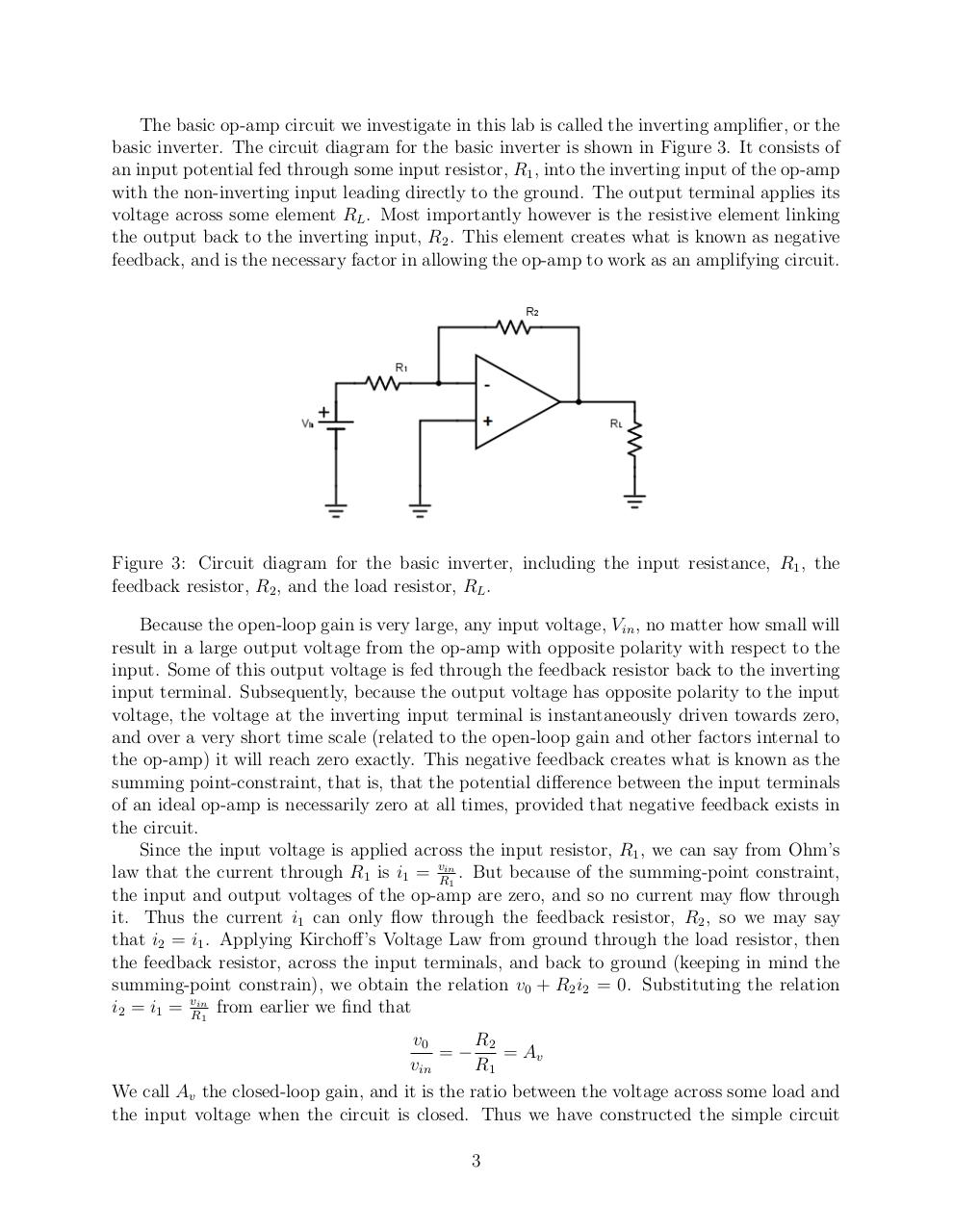# Op Amps.pdfPage 1 2 3 4 5 6 7 8 9 10 11 12 13

#### Text preview

The basic op-amp circuit we investigate in this lab is called the inverting amplifier, or the
basic inverter. The circuit diagram for the basic inverter is shown in Figure 3. It consists of
an input potential fed through some input resistor, R1 , into the inverting input of the op-amp
with the non-inverting input leading directly to the ground. The output terminal applies its
voltage across some element RL . Most importantly however is the resistive element linking
the output back to the inverting input, R2 . This element creates what is known as negative
feedback, and is the necessary factor in allowing the op-amp to work as an amplifying circuit.

Figure 3: Circuit diagram for the basic inverter, including the input resistance, R1 , the
feedback resistor, R2 , and the load resistor, RL .
Because the open-loop gain is very large, any input voltage, Vin , no matter how small will
result in a large output voltage from the op-amp with opposite polarity with respect to the
input. Some of this output voltage is fed through the feedback resistor back to the inverting
input terminal. Subsequently, because the output voltage has opposite polarity to the input
voltage, the voltage at the inverting input terminal is instantaneously driven towards zero,
and over a very short time scale (related to the open-loop gain and other factors internal to
the op-amp) it will reach zero exactly. This negative feedback creates what is known as the
summing point-constraint, that is, that the potential difference between the input terminals
of an ideal op-amp is necessarily zero at all times, provided that negative feedback exists in
the circuit.
Since the input voltage is applied across the input resistor, R1 , we can say from Ohm’s
law that the current through R1 is i1 = vRin1 . But because of the summing-point constraint,
the input and output voltages of the op-amp are zero, and so no current may flow through
it. Thus the current i1 can only flow through the feedback resistor, R2 , so we may say
that i2 = i1 . Applying Kirchoff’s Voltage Law from ground through the load resistor, then
the feedback resistor, across the input terminals, and back to ground (keeping in mind the
summing-point constrain), we obtain the relation v0 + R2 i2 = 0. Substituting the relation
i2 = i1 = vRin1 from earlier we find that
v0
R2
=−
= Av
vin
R1
We call Av the closed-loop gain, and it is the ratio between the voltage across some load and
the input voltage when the circuit is closed. Thus we have constructed the simple circuit
3